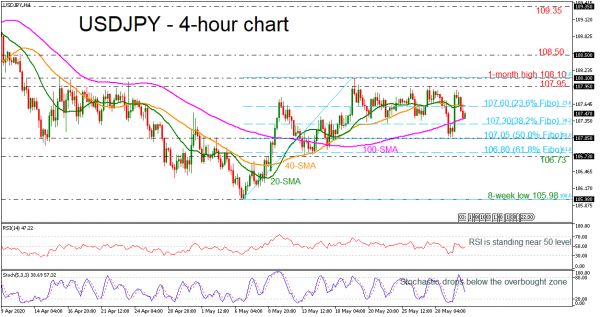USDJPY has reversed back down after finding resistance at slightly below the 108.00 handle. The momentum indicators are pointing to a neutral to negative bias in the short term with the RSI just below 50 and the stochastic oscillator deep below the overbought area.

Further losses below the 100-period SMA should see the 38.2% Fibonacci retracement level of the upward wave from 105.98 to 108.10 at 107.30. A drop below this area would reinforce the bearish structure in the short-term and open the way towards the next key support of 107.50, which is the 50.0% Fibonacci. Below that, the price could reach the 61.8% Fibonacci of 106.80 and the 106.73 support.

In the event of an upside reversal, the 23.6% Fibonacci at 107.60, which overlaps with the 20- and 40-period simple moving averages (SMAs) could act as a barrier before being able to re-challenge the 107.95 and the 108.10 resistances. Further gains could lead the way towards the 108.50 barrier, identified on April 13.